# Maxwell’s Equations-Unification of Electricity & Magnetism: Demystified – Abdul Haque Mohammed

Title:  Maxwell’s Equations-Unification of Electricity & Magnetism: Demystified

Author: Abdul Haque Mohammed, Sr. Assistant Professor, Dept. of ECE,

Abstract: Maxwell’s Equations are four of the most influential equations in all of science: Gauss’s law for electric fields, Gauss’s law for magnetic fields, Faraday’s law, and the Ampere-Maxwell Law. These equations relate electric and magnetic field vectors. In this research paper, each equation and its significance are clearly explained in plain English. Maxwell’s equations form the basis for all modern wireless communications systems including TV, Radio, RADAR, Satellite, Cell phone, Bluetooth and Wireless Internet signals. These equations can be combined to produce the wave equation, the basis for the electromagnetic theory of light. The objective of this paper is to help students, engineers and professionals in this area understand the four Maxwell’s equations and their physical meaning. Maxwell’s equations can be applied only to closed mathematical surfaces called as Gaussian surfaces. Vectors are represented in bold faces to differentiate them from scalars.

Keywords: Electrostatic field; time varying fields; electric charge; MMF, EMF; electric and magnetic flux densities; del or nabla; curl-free field; divergence less field; Gaussian surface

Introduction & Background
In 1864, Sir James Clerk Maxwell (1831-1879) a Scottish Physicist and Mathematician derived a set of equations famously known as Maxwell’s equations. He began his work after reading the papers of previous workers viz. Ampere, Gauss, Helmholtz and Thomson . Maxwell’s equations are also called as equations of electromagnetic waves. When Maxwell worked out his theory of electromagnetism, he ended up with not four but twenty equations that describe the behavior of electric and magnetic fields. It was Oliver Heaviside in Great Britain and Heinrich Hertz in Germany who combined and simplified Maxwell’s equations into four equations in the two decades after Maxwell’s death . Maxwell unified the theory of electricity and magnetism which were originally thought of as two separate aspects. Many physicists consider Maxwell’s equations the most important equations ever devised. Sir Michael Faraday of London discovered electromagnetic induction in the year Maxwell was born but he lacked the mathematics to describe his results quantitatively .With his mathematical genius, Maxwell was able to predict the existence of electromagnetic waves though he didn’t demonstrate it experimentally. He also investigated that light itself is an electromagnetic waves and can therefore travel with a velocity, V = 3×108 m/s. But within 2 decades of his death, Sir Heinrich Hertz, a German Physicist, produced electromagnetic waves in his lab in Germany. In 1909, an Italian inventor, Guglielmo Marconi transmitted these radio waves from England to America. The waves were carrying the letter ‘S’ . A radio wave is an electromagnetic wave in which electric and magnetic fields are at right angles to each other and also to the direction of wave propagation. There can exist in a space a stationary electric field without the presence of a magnetic field in the same space, and alternatively a stationary magnetic field can exist alone without an electric field. However, if the fields are changing it is impossible for either to exist separately; a changing electric field will produce a magnetic field, and a changing magnetic field produces an electric field . From this it is clear that electricity and magnetism essentially are two different aspects of same thing. The electric field which is not created by a stationary charge and that is created as a result of changing magnetic field is called as an induced electric field. Maxwell’s equations involve both electrostatic and induced electric fields. An induced electric field is non-conservative in nature meaning it cannot simply be created from stationary charges unlike static electric field and is therefore independent of potential energy.

Maxwell’s First Equation in Differential Form (or) Gauss’s Law for Electric Fields
Statement: For a charged region, it states that the total charge displaced through a closed surface is equal to the net charge present in the volume surrounded by the surface. Mathematically it is stated as: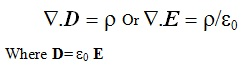Here D is a vector quantity that represents charge displacement density and ρ is volume charge density and can be defined for charged region only. E is electric field and is also a vector. It should be noted that Maxwell’s equations relates fields to their sources that produce them.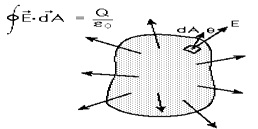Fig: 1. Illustration of Maxwell’s First Equation 

A diverging or converging electric field is produced by a stationary charge . It is one of the Maxwell’s equations for electrostatic fields. For charge free region, it states that the total charge displaced through a closed surface is zero. Mathematically it is stated as below: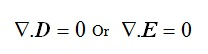Means a diverging or converging field cannot exist in a charge free region.
Maxwell’s Second Equation in Differential form (or) Gauss’s Law for Magnetic Fields
Statement: The total magnetic flux passing through any closed surface is always zero since there is no magnetic charge. Mathematically, it is stated as:Alternatively, we may state it as “The lines of Magnetic flux are continuous and therefore net magnetic flux remaining in a volume is zero”. It means what is entering into a surface is leaving the closed surface. If divergence of a vector field is equal to zero than it is called as divergence less or divergence free field . Magnetic field (B or H) is example of such a field.Fig: 2. Illustration of Maxwell’s second equation. 

Maxwell’s Third Equation in Differential form (or) Faraday’s Law
Statement: It states that EMF around a closed path is equal to time rate of change of flux through the surface enclosed by the path. Alternatively, it states that a circulating electric field is produced by a magnetic field that changes with time.  Such an electric field is called an induced electric field and is independent of any charge/source. Mathematically, it is stated as: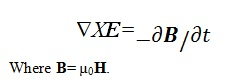Here B is magnetic flux density measured in wb/m2 and H is magnetic field intensity measured in A/m. It should be worth noting that B in magnetic field is similar to D in electric field . This equation shows the relation between time varying electric and magnetic fields. It should be noted that if E in the left hand side of the equation is an electrostatic field than its curl MUST be equal to zero since it is impossible to have a static electric field in space as a result of a time varying magnetic field . Hence this equation can be rewritten for electrostatic field as: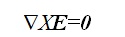From the above equation it can be concluded that a static electric field is curl free or doesn’t have any rotations and is independent of time varying magnetic field but is dependent of charge/source that produces it through the following relation: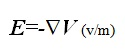where ‘V’ is the scalar electric potential which produces the field. Meaning of this equation is Electric field which is a vector quantity is a negative gradient of electrostatic potential which is a scalar quantity and also that E increases in a direction opposite to V. It can be said that if a vector field such as E can be represented as gradient of a scalar such as V then its curl must be equal to zero. Such a field then is a curl-free field .
Maxwell’s Fourth Equation in Differential form (or) Ampere-Maxwell Law
Statement: It states that MMF around a closed path is equal to sum of displacement current and conduction current through the surface enclosed by the path. Alternatively, it can be stated as follows:
A circulating magnetic field is produced by an electric current and by an electric field that changes with time. 
Mathematically, it is given by: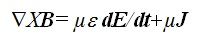Where µ and ε  are constants of media and J is current density measured in A/m2. It is given from Ohm’s law of electrostatic field as: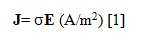For charge free region σ =0 and therefore the second term in the above equation will be zero. Hence the above equation can be rewritten for charge free region as: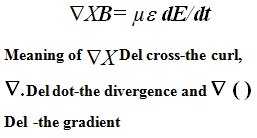The curl of a vector field is a measure of the field’s tendency to circulate about a point-much like the divergence is a measure of the tendency of the field to flow away from or converge into a point . Just as the divergence is found by considering the flux through an infinitesimal surface surrounding the point of interest, the curl at a specified point may be found by considering the circulation per unit area over an infinitesimal path around that point . To understand how Maxwell’s equations lead to the wave equation, it is necessary to understand a third differential operation used in vector calculus-the gradient. Similar to the divergence and the curl, the gradient involves partial derivatives taken in three orthogonal dimensions such as x, y and z. However, whereas the divergence measures the tendency of a vector field to flow away from a point and the curl indicates the circulation of a vector field around a point, the gradient applies to scalar fields . Unlike a vector field, a scalar field is specified entirely by its magnitude at various locations: one example of a scalar field is the height of terrain above sea level. Gradient tells two important things: the magnitude of the gradient indicates how quickly the field is changing over space, and the direction of the gradient indicates the direction in that the field is changing most quickly with distance . The symbol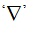del or nabla used throughout is a vector differential operator which shows spatial variations for example it shows variations in x,y, and z directions in a Cartesian coordinate system.

Conclusions
Without electricity and magnetism, it is impossible to imagine modern world where we live. There cannot be motors, generators, lighting, heating, machines and automobiles and modern technologies such as Wireless Internet, Bluetooth, WiMax, RADAR, TV, DAB Radio, and GPS without electricity and magnetism. Also, we cannot imagine modern medical equipment, advances in medical imaging technologies such as MRI technique and development of medical devices and instruments without their existence. In other words, Electricity and Magnetism have revolutionized our World . They are unified and are related through Maxwell’s Equations. It is therefore necessary to understand the relation between electricity and magnetism. In this paper, four of the most influential equations in all of science: The Maxwell’s equations are explained in plain English. This paper can be used by Researchers, System Design Engineers, Scientists and Students working or dealing in the broad area of electronic communications and by anyone as a quick reference material. However, it is beyond the scope of this paper to explain the Maxwell’s Equations in depth. It merely helps in understanding the significance and meanings of various terms involved in such equations and show the interrelations between electric and magnetic field parameters.

References

• Jordan, E.C. and Balmain, K.G. (1968) Electromagnetic Waves and Radiating Systems. 2nd edn. New Jersey: Prentice-Hall, INC.
• Kraus, John D. and Fleisch, Daniel A. (1999) Electromagnetics with applications. 5th edn. United States: McGraw-Hill Intl. Edn.
• Sadiku, Matthew N.O. (2009) Principles of Electromagnetics. 4th edn. International Version. New Delhi: Oxford University Press
• Griffith, David J. (1999) Introduction to Electrodynamics. 3rd edn. New Delhi: Prentice-Hall of India.
• Glazier E.V.D. and Lamont H.R.L. (1958) Transmission and Propagation. London: HMSO. Reprint, Delhi: N. C. Jain.
• Magnetic flux illustrations. Available at: http://hyperphysics.phy-astr.gsu.edu/hbase/magnetic/fluxmg.html (Accessed: 18 Jan 2014)
• Fleisch, Daniel (2008) A Student’s Guide to Maxwell’s Equations. New Delhi: Cambridge University Press
• Gauss’s Law, Integral Form. Available at: http://hydrogen.physik.uni-wuppertal.de/hyperphysics/hyperphysics/hbase/electric/gaulaw.html (Accessed: 18 Jan 2014)
• Fleisch, Dan. (2006) ‘Roots of Telecommunications’, Available at: http://www.youtube.com/watch?v=vPdnTK0fmQU (Accessed: 19 Jan. 2014).